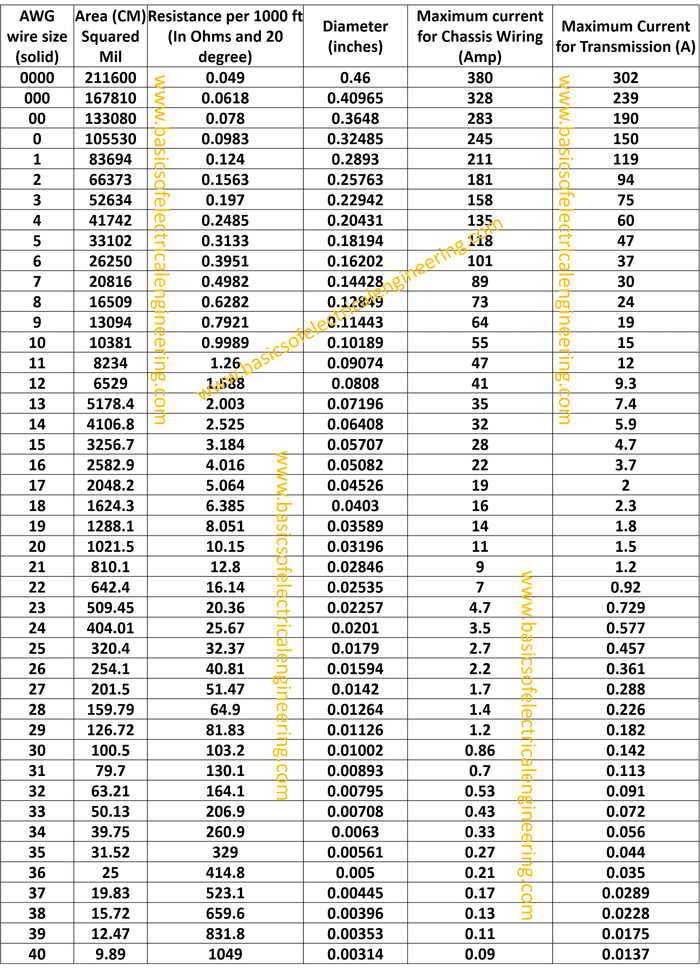# Basics of Electrical Engineering

Learn the basics of Electrical Engineering.

We previously studied about current. But how to determine the diameter of wire for carrying a specific amount of current.

Wire gauge is the measurement of wire diameter. Wire gauge actually explains how much electrical current a wire can safely carry. American Wire Gauge or AWG is a wire table which is the primary system used for selecting wires. Let's have a look at it:Here a few points for using the table.
1. The table is for solid wires. Practically, the wires are stranded (Made of many strands instead of a single solid conductor.
2. For a change of gauge number by 3 the area is doubled (approx). That is for AWG 37 the area is 19.83 whereas for 34 it is 39.76 which is approximately double in number.